101 Concepts for the Level I Exam

# Essential Concept 96: Short-term rates and the business cycleThe price of a default-free nominal coupon-paying bond can be expressed as: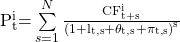where: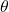= additional return required by investors to compensate for inflation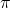= risk premium for uncertainty in future inflation

With short-term nominal interest rates inflation uncertainty can be ignored and short-term T-bills can be priced as:

Short-term nominal interest rates are positively related to short-term real interest rates and short-term inflation expectations. The interest rates are higher in economies with higher inflation and higher, more volatile growth.

Central banks set short-term interest rates in response to the economy’s position in the business cycle.

• They cut rates when economic activity and/or inflation is slow.
• They increase rates when economic activity and/or inflation is high.

The Taylor rule is a rule for setting policy rates and determining whether the rate is at an appropriate level. The rule is given below: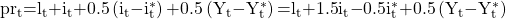where: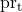= the policy rate at time t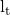= the level of real short-term interest rates that balance long-term savings and borrowing in the economy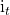= the rate of inflation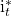= the target rate of inflation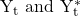= the logarithmic levels of actual and potential real GDP respectively.

A neutral policy rate is the policy rate that neither spurs nor impedes real economic activity.